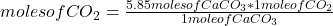## If 5.85 moles of CaCO3 are used in an experiment, how many moles of carbon dioxide are created? (Be sure to include units in your answer.) C

Question

If 5.85 moles of CaCO3 are used in an experiment, how many moles of carbon dioxide are created? (Be sure to include units in your answer.) CaCO3 → CaO + CO2

in progress 0
6 months 2021-08-05T17:04:46+00:00 1 Answers 10 views 0

5.85 moles of carbon dioxide are created.

Explanation:

The balanced reaction is:

CaCO₃ → CaO + CO₂

By stoichiometry of the reaction (that is, the relationship between the amount of reagents and products in a chemical reaction), the following amounts of moles of each compound participate in the reaction:

• CaCO₃: 1 mole
• CaO: 1 mole
• CO₂: 1 mole

Then you can apply the following rule of three: if by stoichiometry 1 mole of CaCO₃ produces 1 mole of CO₂, 5.85 moles of CaCO₃ will produce how many moles of CO₂?moles of CO₂= 5.85

5.85 moles of carbon dioxide are created.